# RD Sharma Solutions for Class 8 Maths Chapter 3 - Squares and Square Roots Exercise 3.8

Students can refer and download the RD Sharma Solutions for the Exercise 3.8 of Class 8 Maths Chapter 3, Squares and Square roots from the link available here. Our experts have solved the RD Sharma Solutions to ensure that the students are thorough with their concepts by practising the solutions. Exercise 3.8 of Class 8 is about finding the approximate values of square roots by the method of long division. Students are advised to practice the RD Sharma Solutions Class 8 for better understanding of the concepts, which in turn helps in securing high marks in their examination.

## Download the pdf of RD Sharma Solutions For Class 8 Maths Chapter 3 Squares and Square Roots Exercise 3.8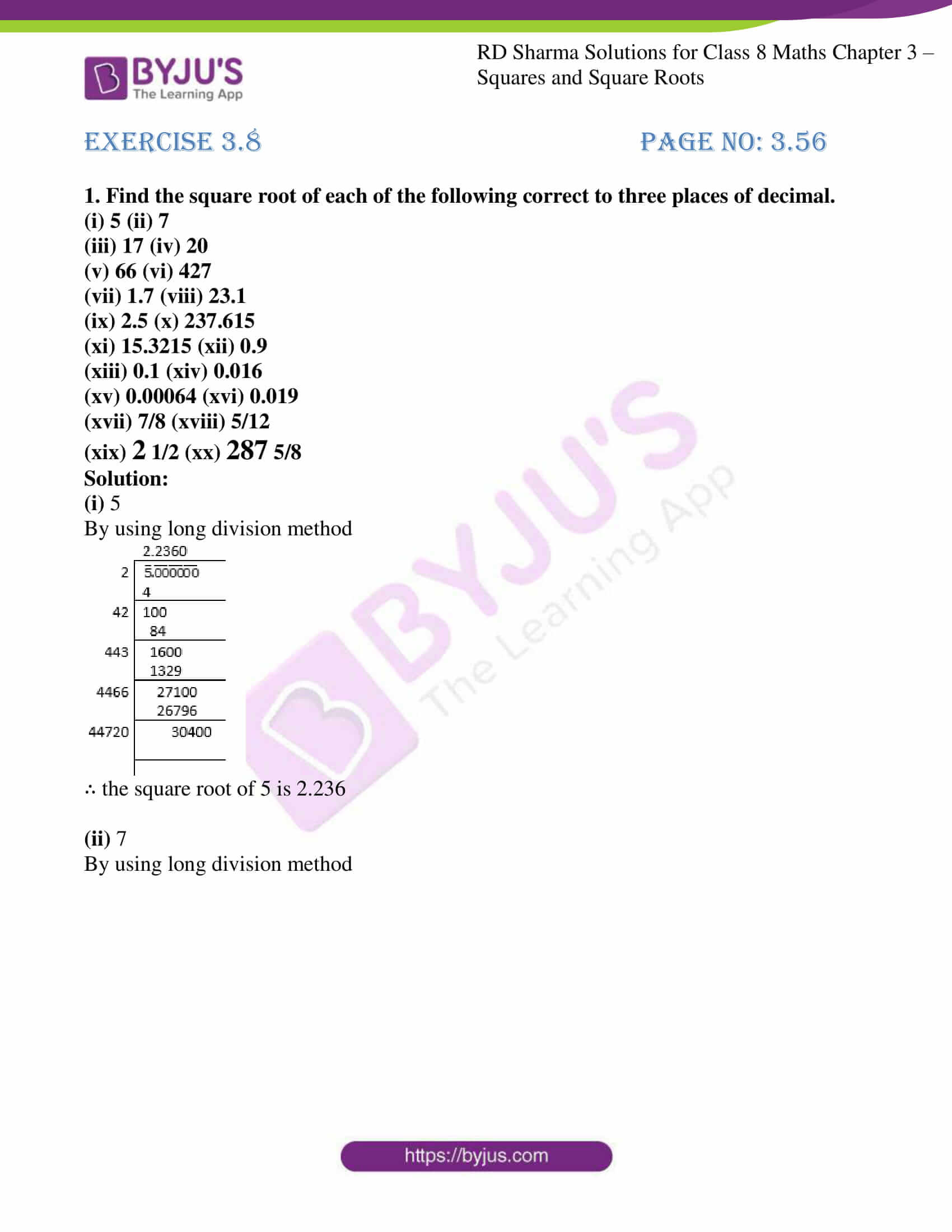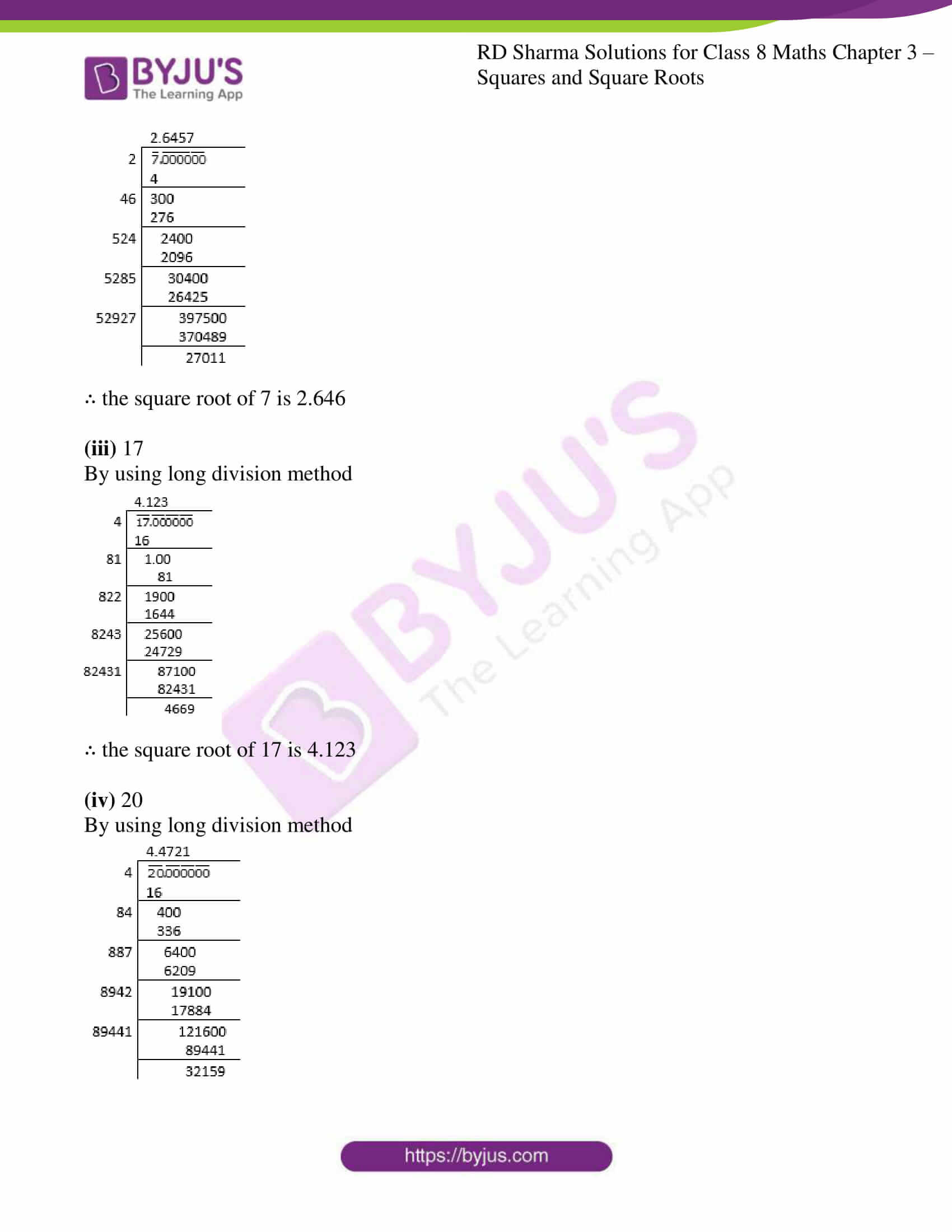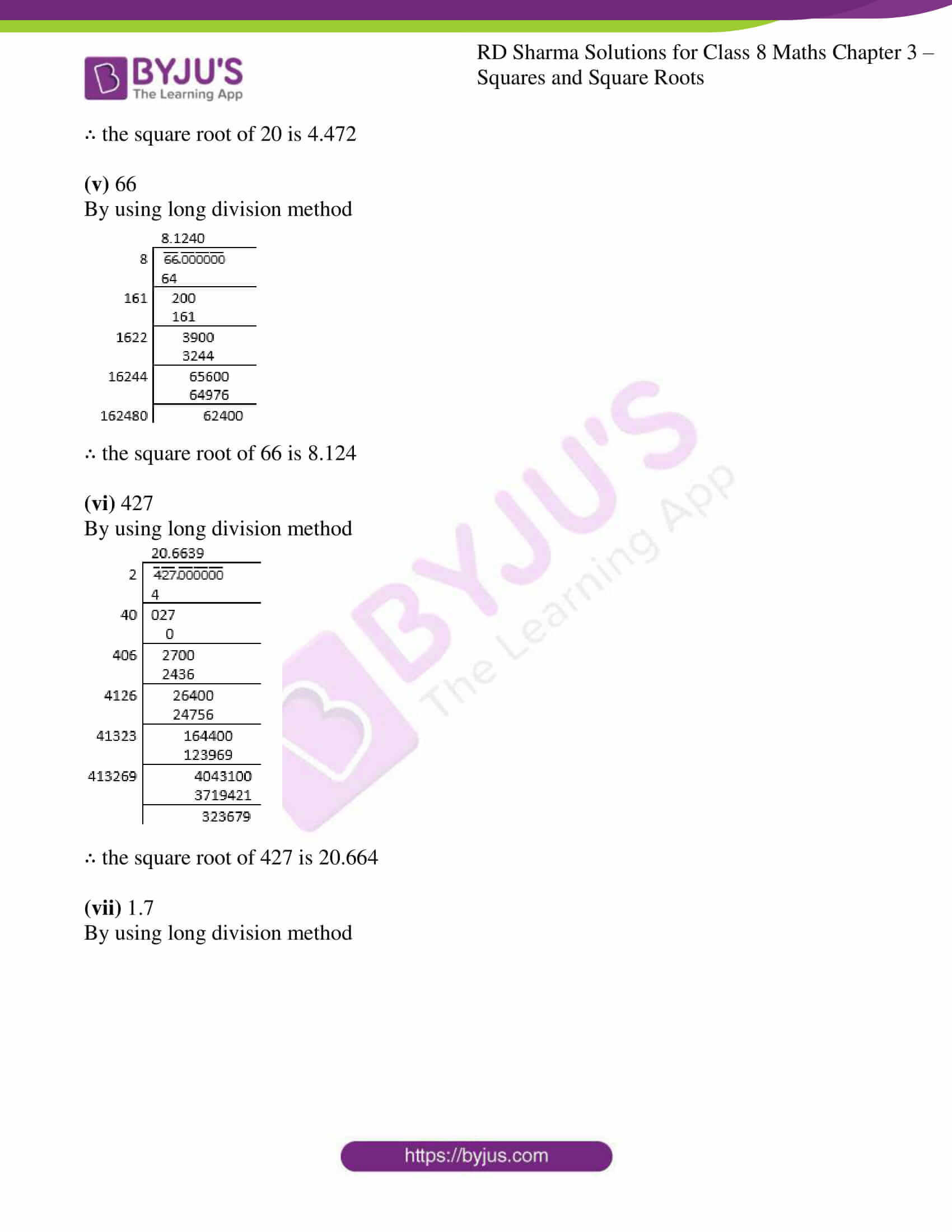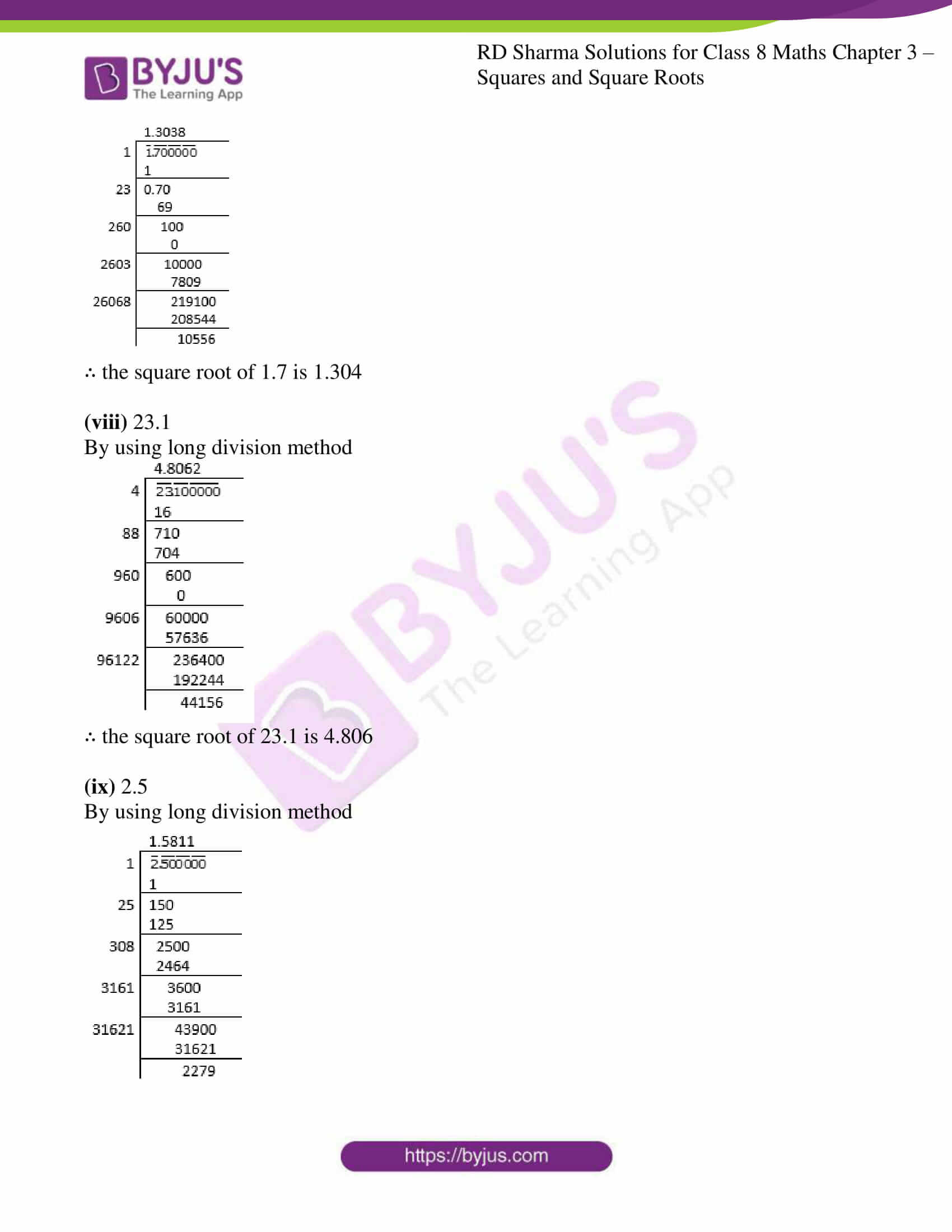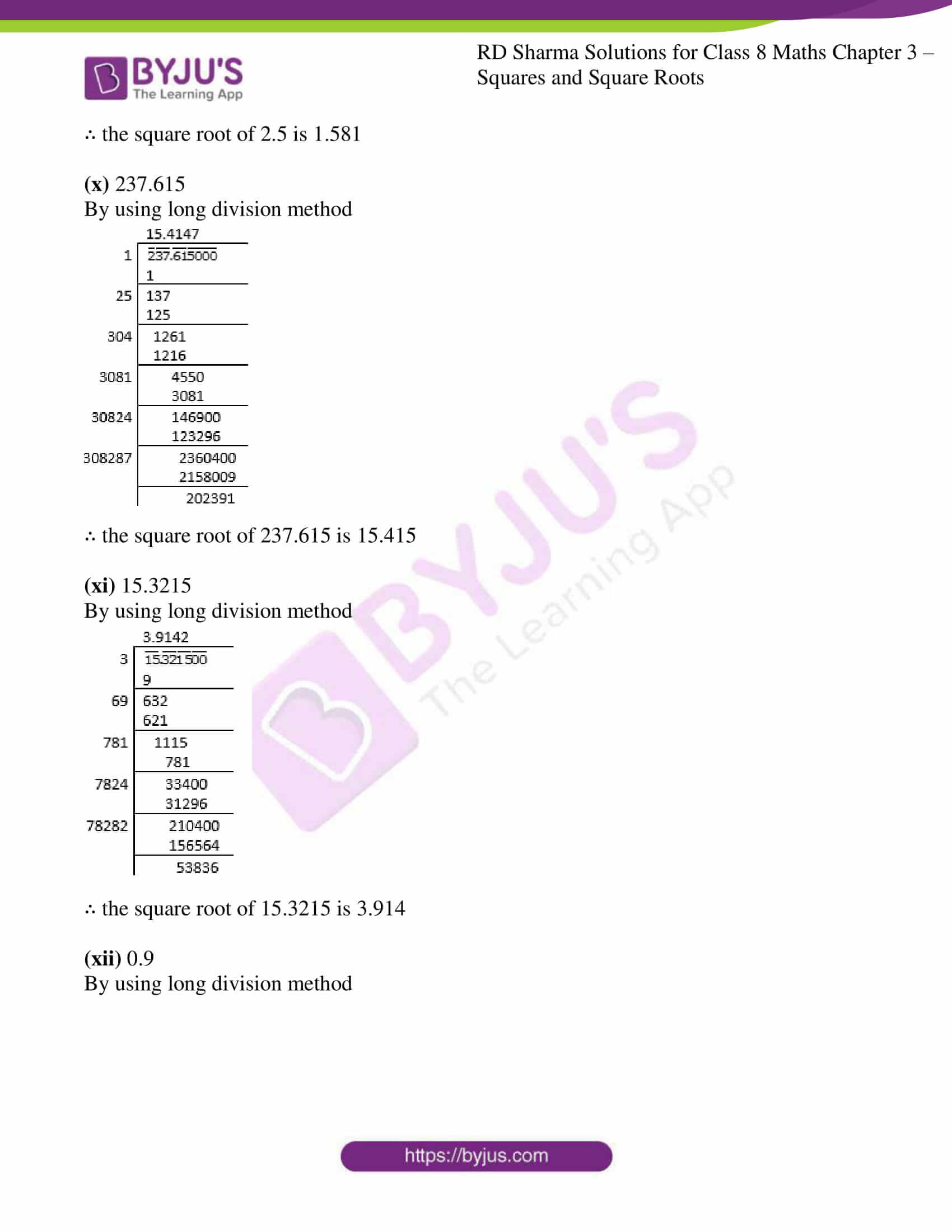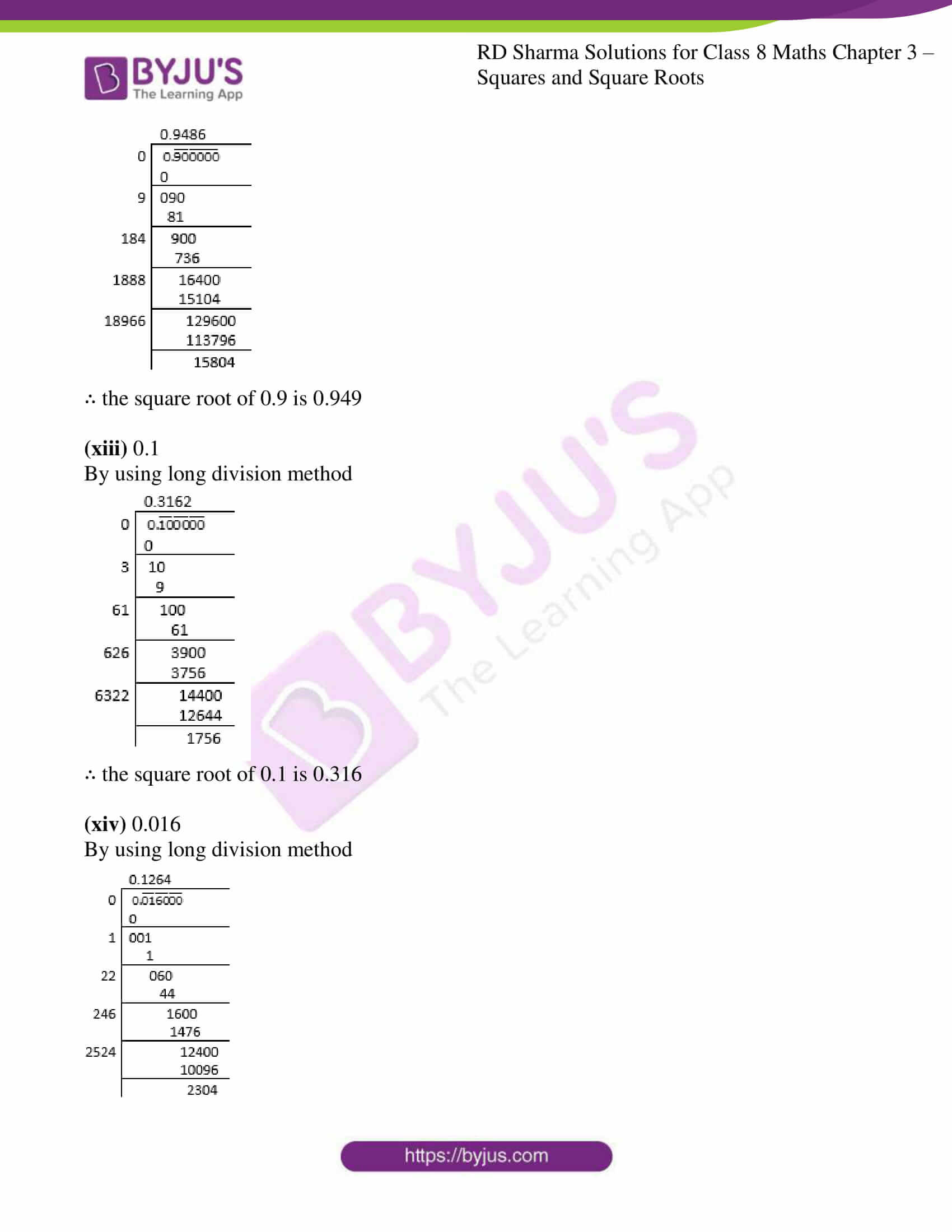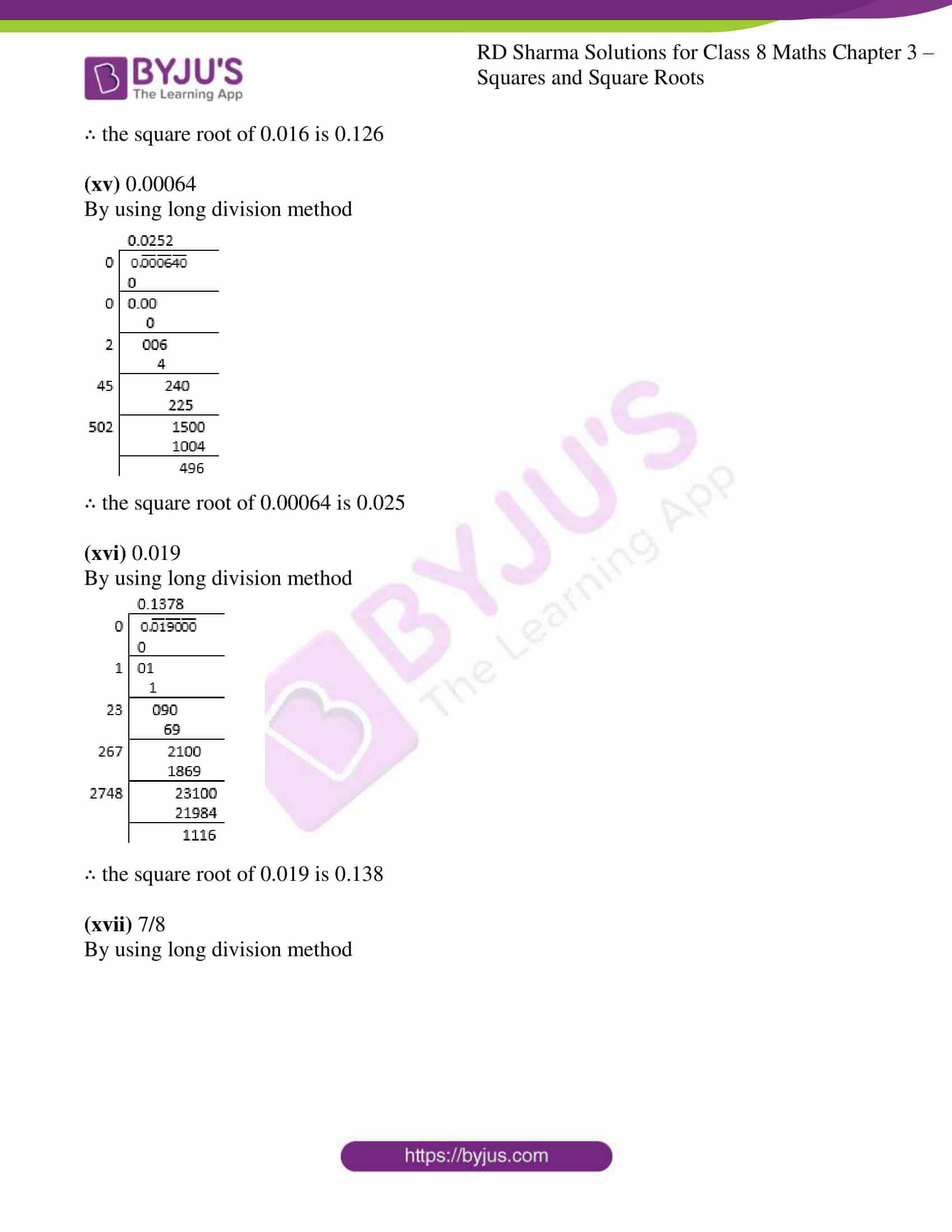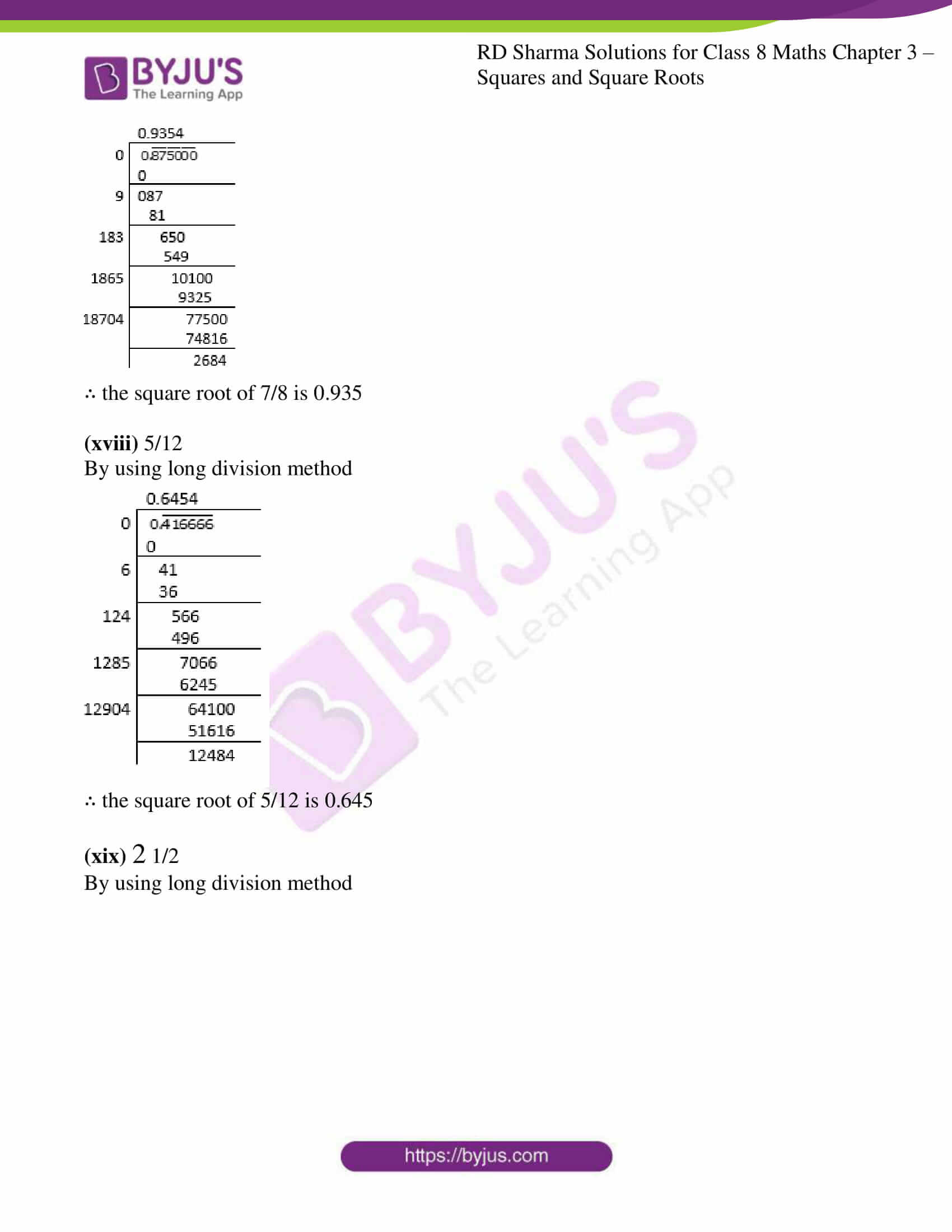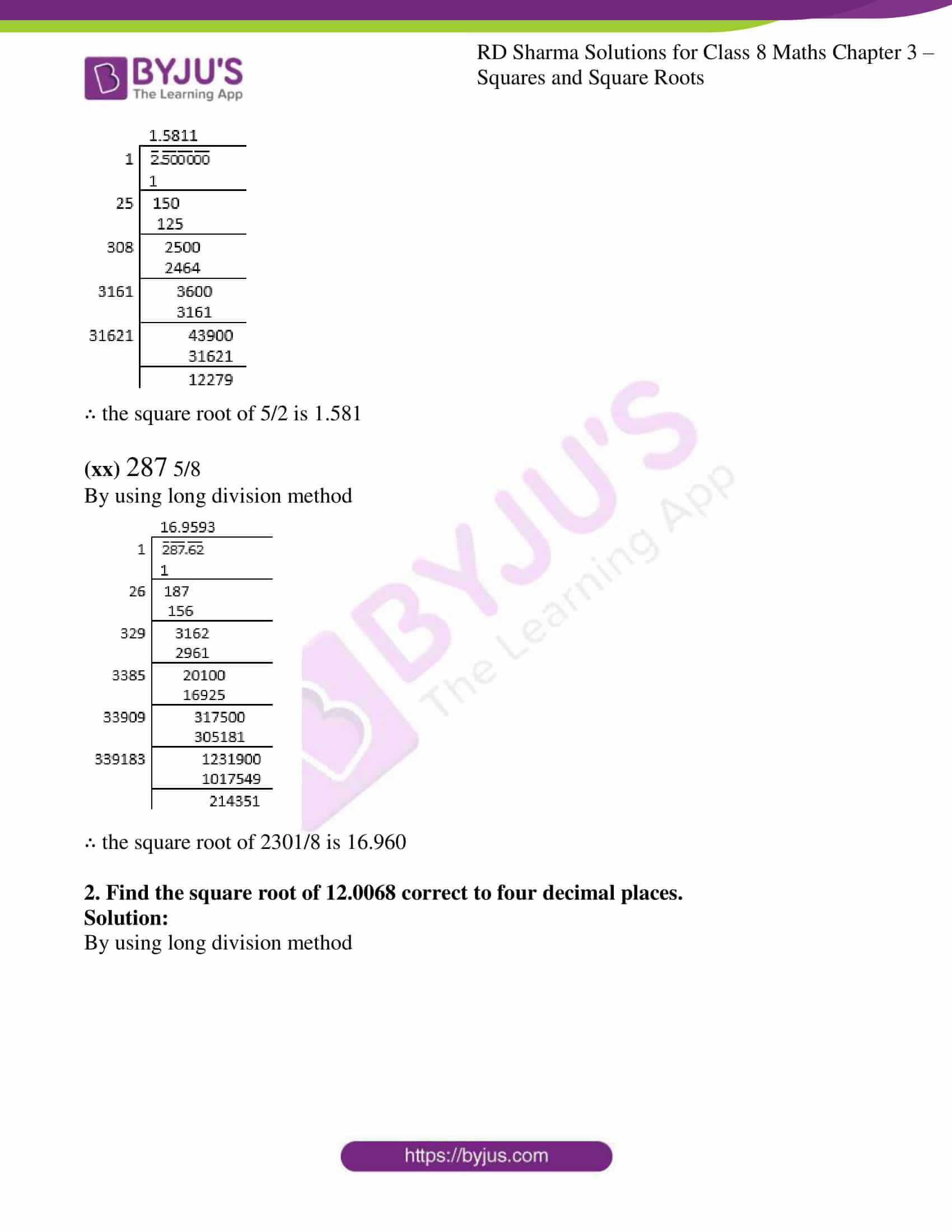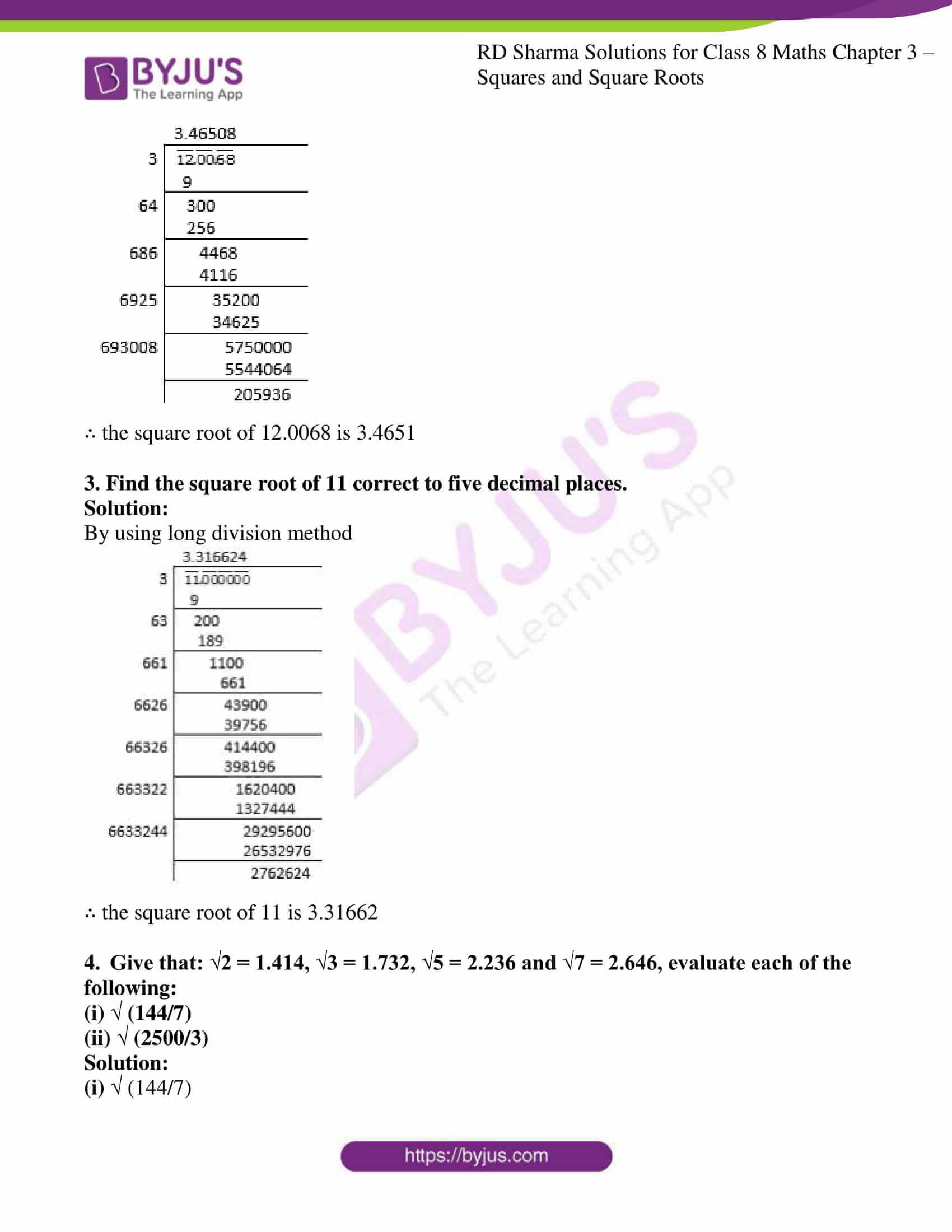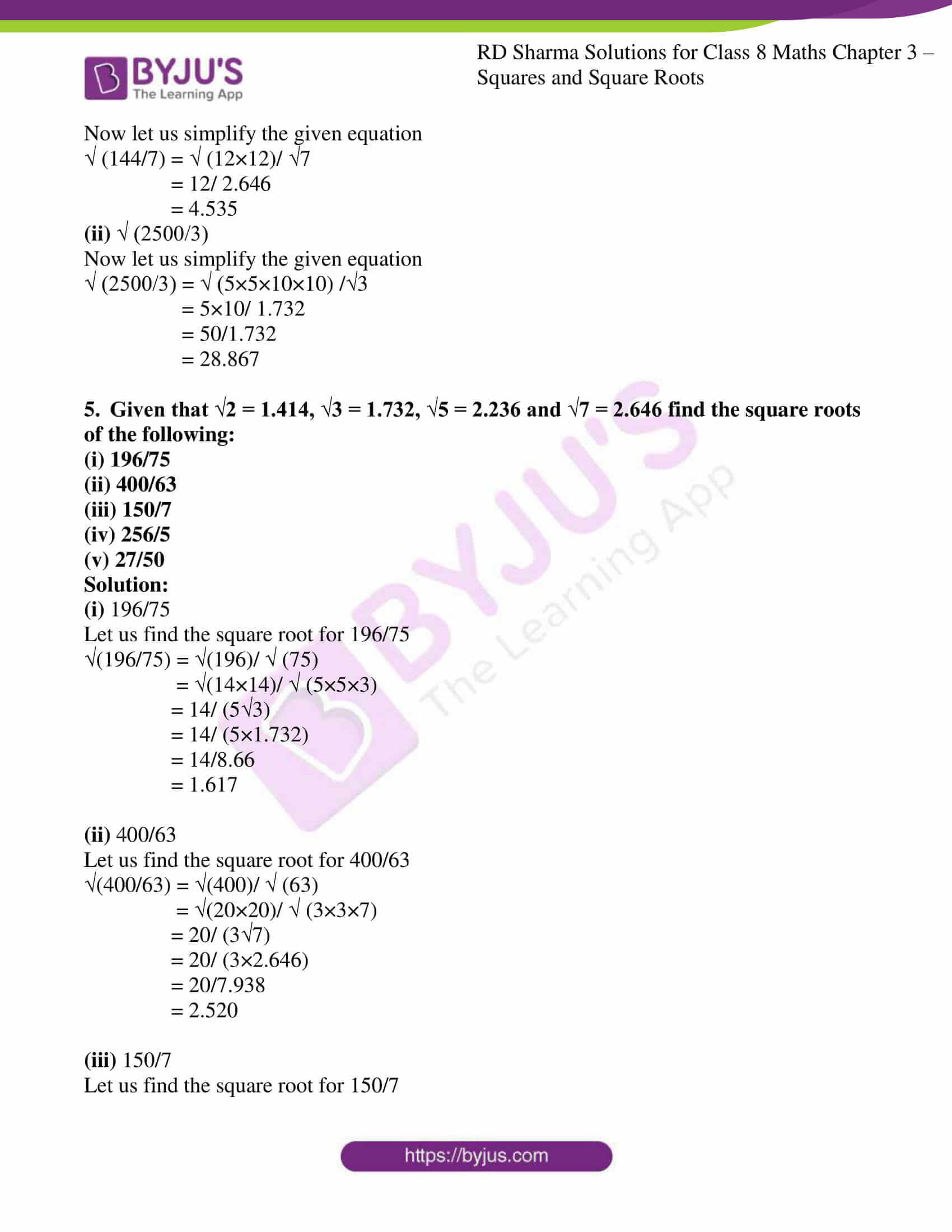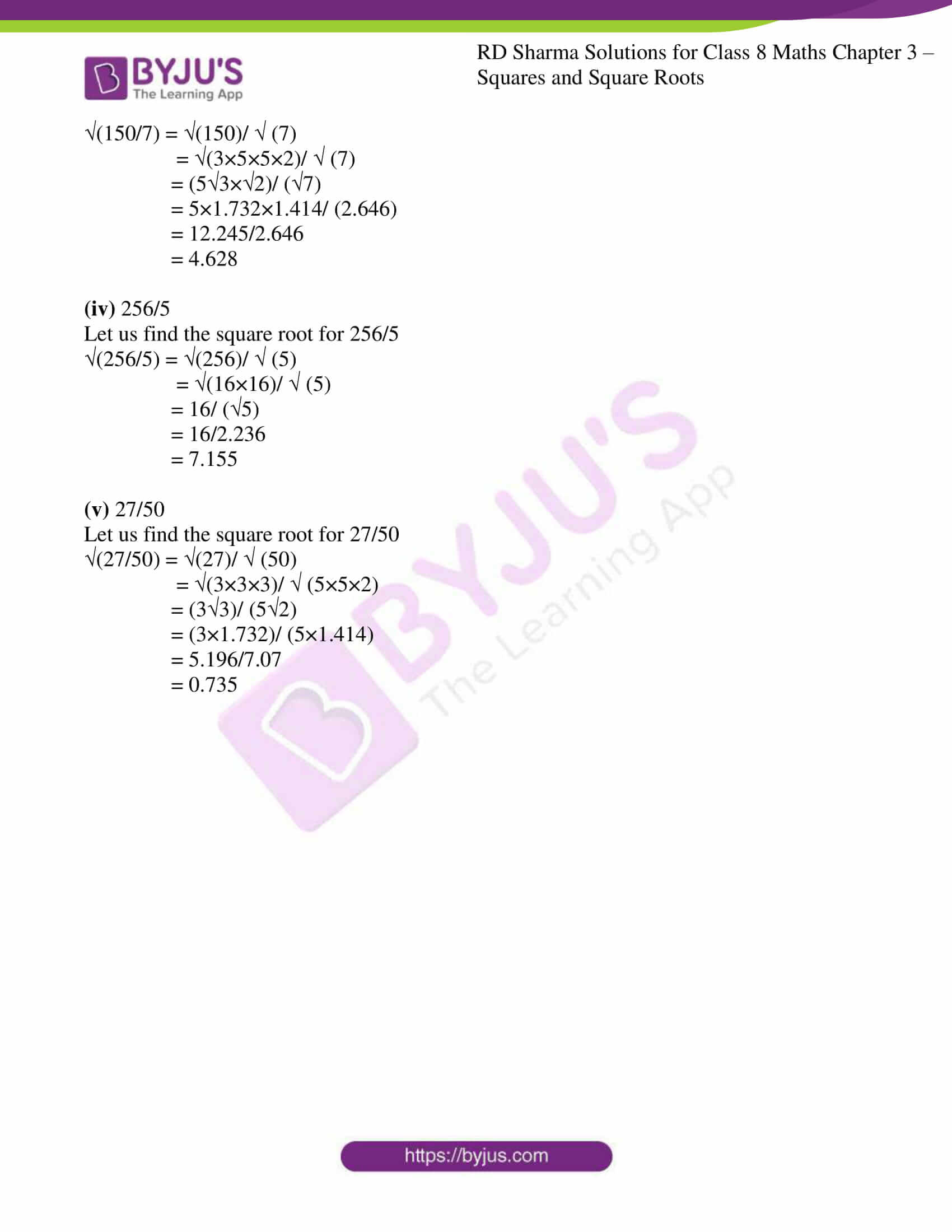### Access Answers to RD Sharma Solutions for Class 8 Maths Exercise 3.8 Chapter 3 Squares and Square Roots

#### EXERCISE 3.8 PAGE NO: 3.56

1. Find the square root of each of the following correct to three places of decimal.
(i) 5 (ii) 7
(iii) 17 (iv) 20
(v) 66 (vi) 427
(vii) 1.7 (viii) 23.1
(ix) 2.5 (x) 237.615
(xi) 15.3215 (xii) 0.9
(xiii) 0.1 (xiv) 0.016
(xv) 0.00064 (xvi) 0.019
(xvii) 7/8 (xviii) 5/12
(xix) 2 1/2 (xx) 287 5/8

Solution:

(i) 5

By using long division method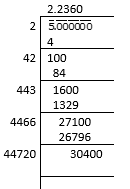∴ the square root of 5 is 2.236

(ii) 7

By using long division method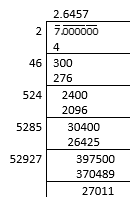∴ the square root of 7 is 2.646

(iii)
17

By using long division method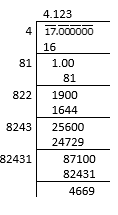∴ the square root of 17 is 4.123

(iv) 20

By using long division method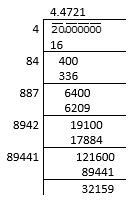∴ the square root of 20 is 4.472

(v)
66

By using long division method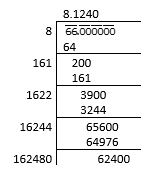∴ the square root of 66 is 8.124

(vi) 427

By using long division method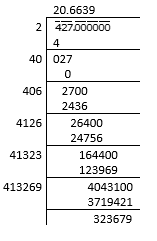∴ the square root of 427 is 20.664

(vii)
1.7

By using long division method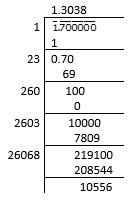∴ the square root of 1.7 is 1.304

(viii) 23.1

By using long division method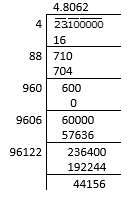∴ the square root of 23.1 is 4.806

(ix)
2.5

By using long division method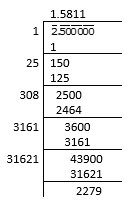∴ the square root of 2.5 is 1.581

(x) 237.615

By using long division method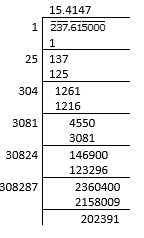∴ the square root of 237.615 is 15.415

(xi)
15.3215

By using long division method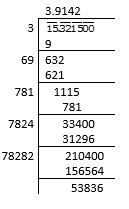∴ the square root of 15.3215 is 3.914

(xii) 0.9

By using long division method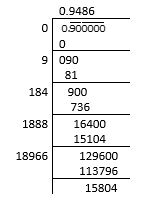∴ the square root of 0.9 is 0.949

(xiii)
0.1

By using long division method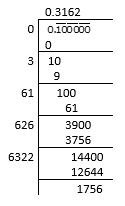∴ the square root of 0.1 is 0.316

(xiv) 0.016

By using long division method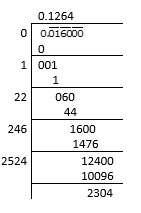∴ the square root of 0.016 is 0.126

(xv)
0.00064

By using long division method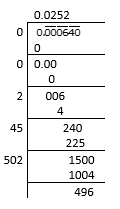∴ the square root of 0.00064 is 0.025

(xvi) 0.019

By using long division method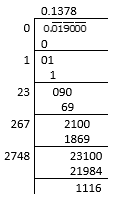∴ the square root of 0.019 is 0.138

(xvii)
7/8

By using long division method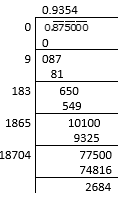∴ the square root of 7/8 is 0.935

(xviii) 5/12

By using long division method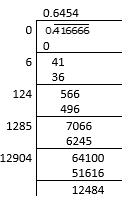∴ the square root of 5/12 is 0.645

(xix)
2 1/2

By using long division method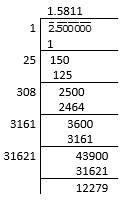∴ the square root of 5/2 is 1.581

(xx) 287 5/8

By using long division method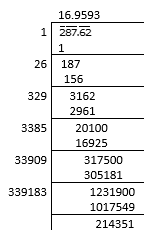∴ the square root of 2301/8 is 16.960

2. Find the square root of 12.0068 correct to four decimal places.

Solution:

By using long division method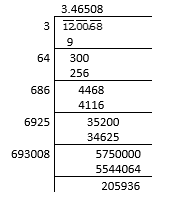∴ the square root of 12.0068 is 3.4651

3. Find the square root of 11 correct to five decimal places.

Solution:

By using long division method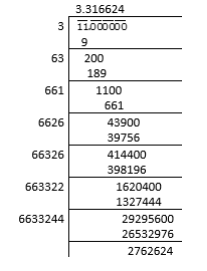∴ the square root of 11 is 3.31662

4. Give that: √2 = 1.414, √3 = 1.732, √5 = 2.236 and √7 = 2.646, evaluate each of the following:
(i) √ (144/7)
(ii) √ (2500/3)

Solution:

(i) √ (144/7)

Now let us simplify the given equation

√ (144/7) = √ (12×12)/ √7

= 12/ 2.646

= 4.535

(ii) √ (2500/3)

Now let us simplify the given equation

√ (2500/3) = √ (5×5×10×10) /√3

= 5×10/ 1.732

= 50/1.732

= 28.867

5. Given that √2 = 1.414, √3 = 1.732, √5 = 2.236 and √7 = 2.646 find the square roots of the following:
(i) 196/75
(ii) 400/63
(iii) 150/7
(iv) 256/5
(v) 27/50

Solution:

(i) 196/75

Let us find the square root for196/75

(196/75) = (196)/ (75)

= (14×14)/ (5×5×3)

= 14/ (53)

= 14/ (5×1.732)

= 14/8.66

= 1.617

(ii)
400/63

Let us find the square root for400/63

(400/63) = (400)/ (63)

= (20×20)/ (3×3×7)

= 20/ (37)

= 20/ (3×2.646)

= 20/7.938

= 2.520

(iii) 150/7

Let us find the square root for150/7

(150/7) = (150)/ (7)

= (3×5×5×2)/ (7)

= (52)/ (7)

= 5×1.732×1.414/ (2.646)

= 12.245/2.646

= 4.628

(iv) 256/5

Let us find the square root for256/5

(256/5) = (256)/ (5)

= (16×16)/ (5)

= 16/ (5)

= 16/2.236

= 7.155

(v)
27/50

Let us find the square root for27/50

(27/50) = (27)/ (50)

= (3×3×3)/ (5×5×2)

= (33)/ (52)

= (3×1.732)/ (5×1.414)

= 5.196/7.07

= 0.735

## RD Sharma Solutions for Class 8 Maths Exercise 3.8 Chapter 3 – Squares and Square Roots

Exercise 3.8 of RD Sharma Solutions for Chapter 3 Squares and Square Roots, this exercise mainly deals with the concepts related to the long division method to find the approximate values of square roots. The RD Sharma Solutions can help the students practice and learn the fundamentals as it provides all the answers to the questions from the RD Sharma textbook. Practising as many times as possible helps in a better understanding of the concept and also helps in effective time management skills. It also boosts the confidence level to achieve high marks.